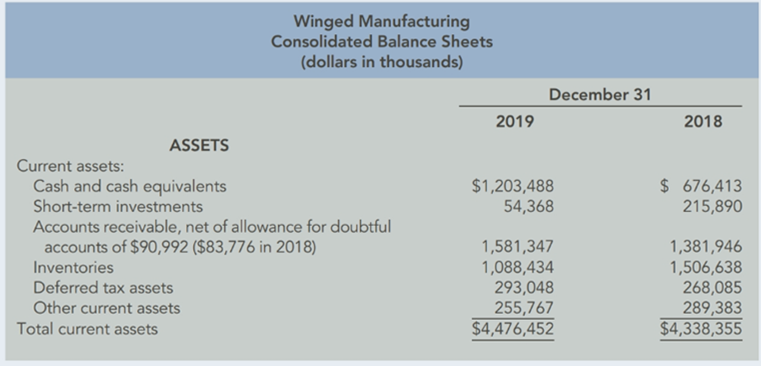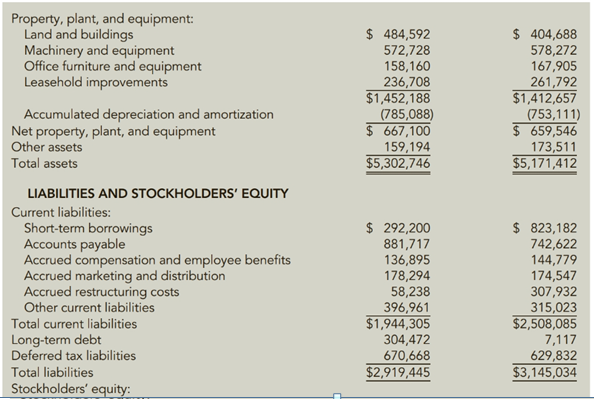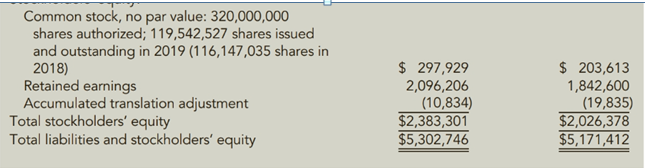Chapter 12, Problem 73E### Cornerstones of Financial Accounti...

4th Edition
Jay Rich + 1 other
ISBN: 9781337690881

#### Solutions

Chapter
Section### Cornerstones of Financial Accounti...

4th Edition
Jay Rich + 1 other
ISBN: 9781337690881
Textbook Problem
3 views

# Vertical Analysis of Balance SheetsConsolidated balance sheets for Winged Manufacturing follow.Required:1. Prepare common size balance sheets for vertical analysis (in percentage terms. rounded to two decimal places). You do not need to include the actual dollar amounts shown above.2. Indicate from what sources Vinged appears to have secured the resources for its asset increase.

To determine

(a)

Introduction:

Vertical financial statements calculate the percentage as the percentage of the largest value on the financial statement

To prepare:

Common size using vertical analysis in the books of Winged manufacturing.

Explanation

Total of Asset and total of liabilities is taken as a base to calculate the percentage for both the years.

For eg. Percentage of cash and cash equivalents fo

To determine

(b)

To state:

The sources from which winged have secured the resources for its asset increase.

### Still sussing out bartleby?

Check out a sample textbook solution.

See a sample solution

#### The Solution to Your Study Problems

Bartleby provides explanations to thousands of textbook problems written by our experts, many with advanced degrees!

Get Started